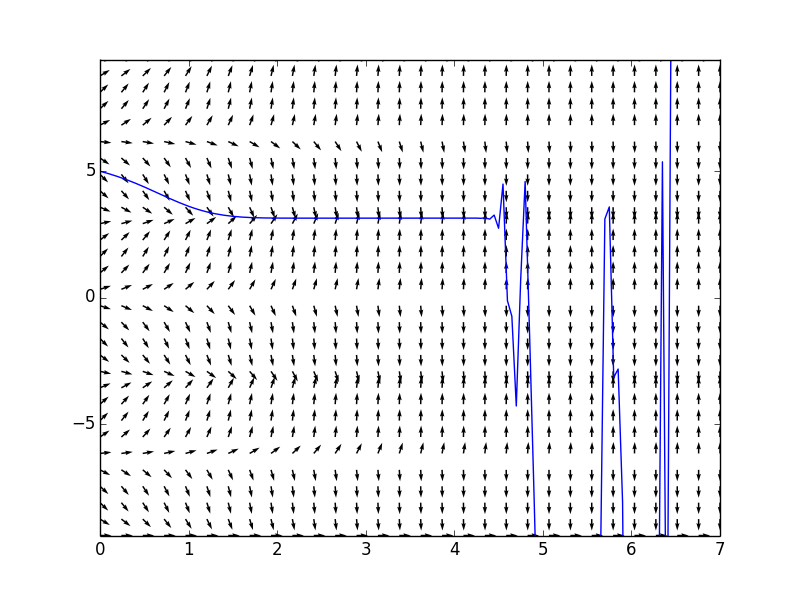# 微分方程解的存在性和唯一性

## 微分方程的定义域

$frac{dy}{dt}=f(t,y)$

$frac{dy}{dt} = y^3+t^2$
$frac{dy}{dt} = y^2$

$frac{dy}{dt} = frac{y}{t}$

## 存在性定理：

$frac{dy}{dt}=1+y^2,qquad y(0) = 0$

## 唯一性定理

$frac{dy}{dt}=sqrt{y}sin(2t), qquad y(0)=0$

1. 蓝色（平衡解）：
$y_1(t) = 0, forall t in mathbb{R}$
2. 黑色：
$y_2(t) = sqrt{frac{8}{27}}sin^3t$
3. 红色：
$y_2(t) = -sqrt{frac{8}{27}}sin^3t$$frac{partial f}{partial y}= frac{sin(2t)}{{3y}^{frac{2}{3}}}$
y不可取0，因而不是连续的，因此违背唯一性定理。

## 例子

$frac{dy}{dt}=-2ty^2,frac{partial f}{partial y}=-4ty$$frac{dy}{dt}=e^{t}siny,qquad y(0)=5$